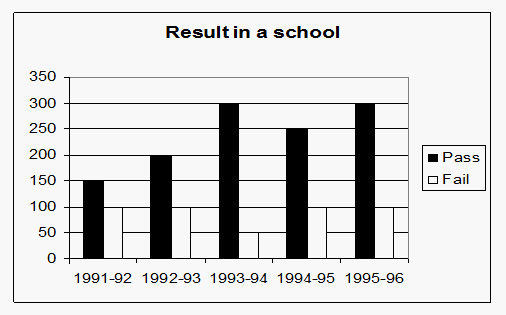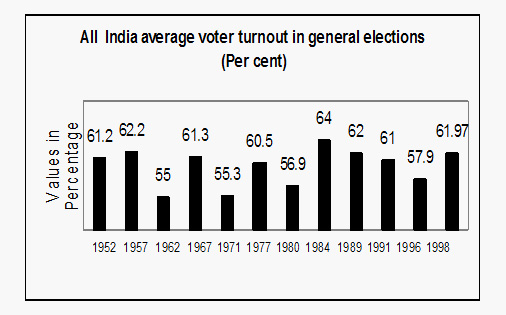# Bar Graph Questions: Level 02

Directionsfor questions 1 - 5: Study the following graph carefully and answer the questions that follow. The graph shows the results of students in a school.1. The difference of number of students passed to those failed is minimum in which year?
A. 1991-92
B. 1992-93
C. 1993-94
D. 1994-95
2. How many times number of failed students is same?
A. 1
B. 2
C. 3
D. 4
3. What is an approximate percentage of students failed during 5 years?
A. 65%
B. 27%
C. 51%
D. 80%
4.  When is the percentage increase in the total number of students maximum in comparison to the previous year?
A. 1995-96
B. 1994-95
C. 1993-94
D. 1992-93
5. What is an average number of students failed in the school in last 5 years?
A. 190
B. 180
C. 80
D. 90
Directions for questions 6 – 10: Study the following graph and answer the questions given below:1. The highest and lowest ever voters turn- out (in percentage)is respectively in which years?
A. 1984 and 1962
B. 1971 and 1977
C. 1957 and 1962
D. 1984 and 1971
2. In which of the options, the numeric difference in voters turnout (in percentage) was nearly equal to 7%?
A. 1957-62
B. 1984-89
C. 1996-98
D. 1971-77
3. The average voter turnout (in percentage) between 1952 to 1998 was approximately?
A. 56.8%
B. 40.9%
C. 62.7%
D. 60%
4. In which year was the growth in the average voter turnout (in percentage) to be the second highest in comparison to the previous elections?
A. 1967
B. 1984
C. 1998
D. 1977
5. Which year had the highest average voters turnout  (in percentage) in comparison to the total no. of voters?
A. 1971
B. 1996
C. 1984